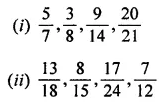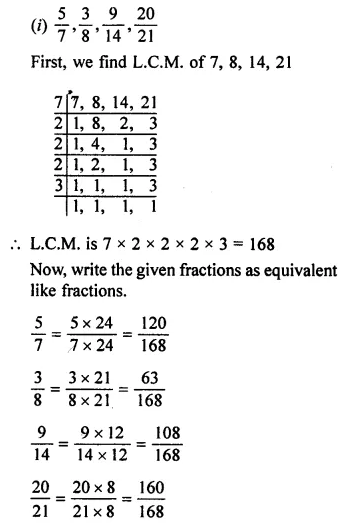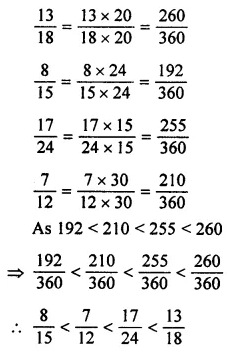# ML Aggarwal Class 7 Solutions for ICSE Maths Chapter 2 Fractions and Decimals Ex 2.1

## ML Aggarwal Class 7 Solutions for ICSE Maths Chapter 2 Fractions and Decimals Ex 2.1

Question 1.
What fraction of each of the following figure is shaded?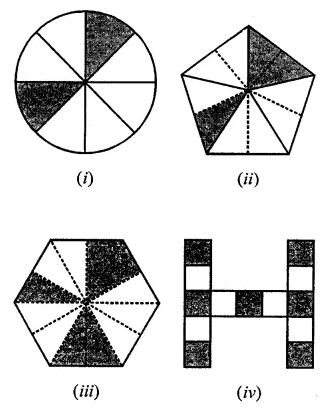Solution:Question 2.
What fraction of an hour is 35 minutes?
Solution: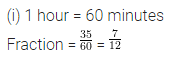Question 3.
Convert the following fractions into improper fractions :
(i) 2$$\frac { 7 }{ 9 }$$
(ii) 5$$\frac { 4 }{ 11 }$$
Solution: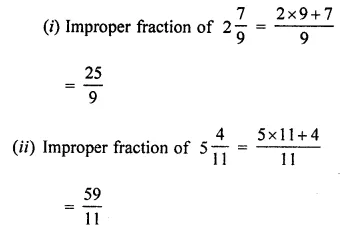Question 4.
Convert the following fractions into mixed fractions:
(i) $$\frac { 73 }{ 8 }$$
(ii) $$\frac { 94 }{ 13 }$$
Solution: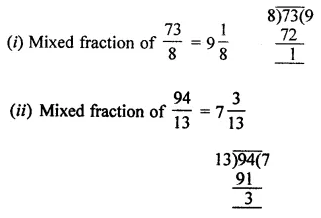Question 5.
Fill in the missing numbers in the following equivalent fractions:
(i) $$\frac { 3 }{ 7 }$$ = $$\frac { …. }{ 35 }$$
(ii) $$\frac { 5 }{ …. }$$ = $$\frac { 30 }{ 18 }$$
(iii) $$\frac { ….. }{ 9 }$$ = $$\frac { 56 }{ 72 }$$
Solution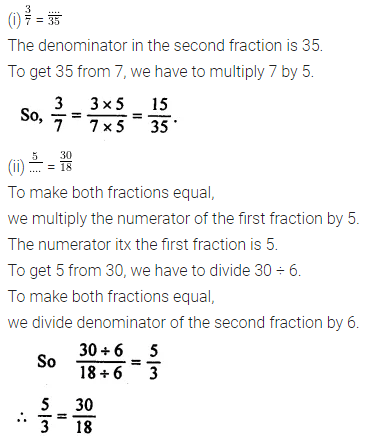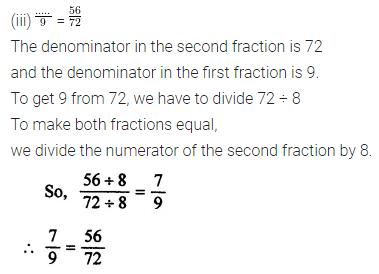Question 6.
Reduce the following fractions to their simplest form:
(i) $$\frac { 48 }{ 72 }$$
(ii) $$\frac { 276 }{ 115 }$$
(iii) $$\frac { 72 }{ 336 }$$
Solution: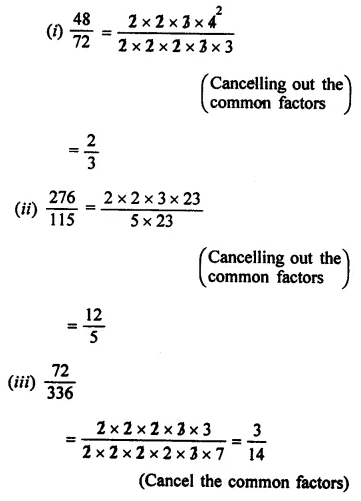Question 7.
Convert the following fractions into equivalent like fractions:Solution: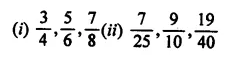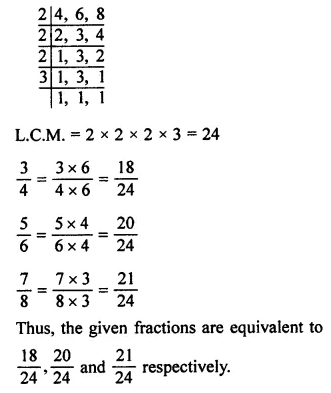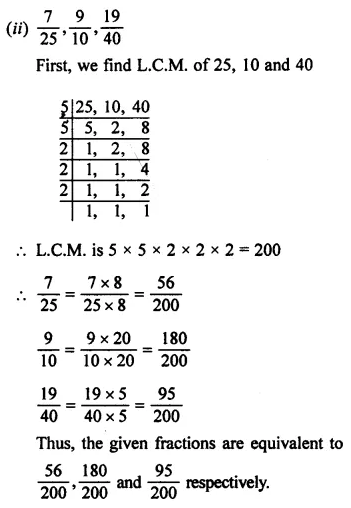Question 8.
Arrange the given fractions in descending order:Solution: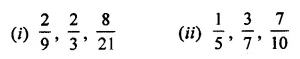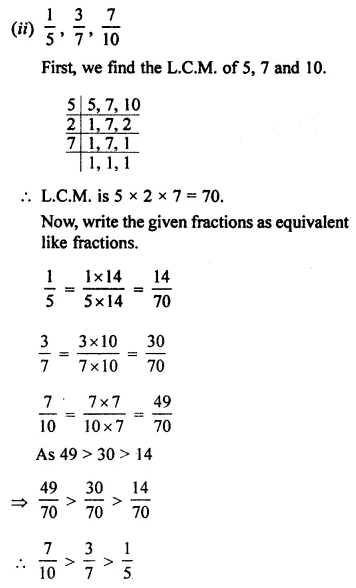Question 9.
Arrange the given fractions in ascending order: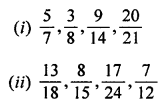Solution: